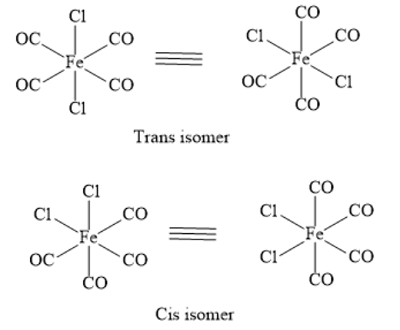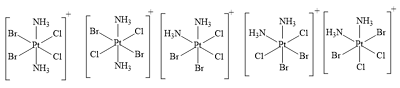# How many geometric isomers are there for each species? a. [Fe(CO)_4 Cl_2]. b....

## Question:

How many geometric isomers are there for each species?

a. {eq}\rm \left [Fe(CO)_4 Cl_2\right ] {/eq}.

b. {eq}\rm \left [Pt(NH_3)_2Cl_2Br_2\right ]^+ {/eq}.

## Geometric Isomers:

The substituents of isomers can be oriented in a variety of ways. They can be identified by two segments:

• "Cis-isomer"
• "Trans-isomer"

The isomers of {eq}\left[ {{\rm{Fe}}{{\left( {{\rm{CO}}} \right)}_4}{\rm{C}}{{\rm{l}}_2}} \right] {/eq} are depicted as:Here, two geometrical isomers are obtained; cis isomers and trans-isomers.

The isomers of {eq}{\left[ {{\rm{Pt}}{{\left( {{\rm{N}}{{\rm{H}}_3}} \right)}_2}{\rm{C}}{{\rm{l}}_2}{\rm{B}}{{\rm{r}}_2}} \right]^ + } {/eq} are appeared as:Here, five geometrical isomers are obtained.Geometric Isomers: Definition & Examples

from

Chapter 1 / Lesson 6
18K

In chemistry, geometric isomers are a condition where the atoms in two molecules (isomers) are connected in the same order, but the resulting shape of the molecule is different. Learn more about the definition of geometric isomers, double bonds, and ring structures through helpful examples.